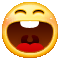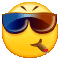# 48.不速之客

VIP畅听

## 用户评论(211)展示条数：20条20条50条100条1！嗯嗯嗯嗯嗯嗯嗯嗯嗯嗯嗯嗯嗯嗯嗯嗯嗯嗯嗯嗯嗯嗯嗯嗯嗯嗯嗯嗯嗯嗯嗯嗯嗯嗯嗯嗯嗯嗯嗯嗯嗯嗯嗯嗯嗯嗯嗯嗯嗯嗯嗯嗯嗯嗯嗯嗯嗯嗯嗯嗯嗯嗯嗯嗯嗯嗯嗯嗯嗯嗯嗯嗯嗯嗯嗯嗯嗯嗯嗯嗯嗯嗯嗯嗯嗯嗯嗯嗯嗯嗯嗯嗯嗯嗯嗯嗯嗯嗯嗯嗯嗯嗯嗯嗯嗯嗯嗯嗯嗯嗯嗯嗯嗯嗯嗯嗯嗯嗯嗯嗯嗯嗯嗯嗯嗯嗯嗯嗯嗯嗯嗯嗯嗯嗯嗯嗯嗯嗯嗯嗯嗯嗯嗯嗯嗯嗯嗯嗯嗯嗯嗯嗯嗯嗯嗯嗯嗯嗯嗯嗯嗯嗯嗯嗯嗯嗯嗯嗯嗯嗯嗯嗯嗯嗯嗯嗯嗯嗯嗯嗯嗯嗯嗯嗯嗯嗯嗯嗯嗯嗯嗯嗯嗯嗯嗯嗯嗯嗯嗯嗯嗯嗯嗯嗯嗯嗯嗯嗯嗯嗯嗯嗯嗯嗯嗯嗯嗯嗯嗯嗯嗯嗯嗯嗯嗯嗯嗯嗯嗯嗯嗯嗯嗯嗯嗯嗯嗯嗯嗯嗯嗯嗯嗯嗯嗯嗯嗯嗯嗯嗯嗯嗯嗯嗯嗯嗯嗯嗯嗯嗯嗯嗯嗯嗯嗯嗯嗯嗯嗯嗯嗯嗯嗯嗯嗯嗯嗯嗯嗯嗯嗯嗯嗯嗯嗯嗯嗯嗯嗯嗯嗯嗯嗯嗯嗯嗯嗯嗯

xcw1122

Q Q Q Q Q Q Q Q Wow Wow Q Wow Q Q Q Wow Q Wow Q Q Q Q Q Q Q Q Q Q Q Q Q Q Q W Q Q Q Q W W Q Wow Q Q Q Q Q Q Q Q Q Q Q W W Wow W W Q W W W W W W W W W Q Wow Q Q Q Q Q W W Q W W We Q Wow Q Q Q Wow Q Q Q Wow Q We Q Q Q Q Q Wow Q Q Q Wow Q We Q Q Q Q Wow Q Q Q Q Q Q Q Q Q Q 恭喜你浪费时间